## Saturday, September 19, 2015

### Update to rateshift method that results in much better optimization for models with multiple shifts

I have been working on the phytools function `rateshift`, for locating one or multiple temporal shifts in the rate of evolution for a continuous character on the tree.

This function has nice `print` and `plot` methods, and would seem to be quite nice - however it suffers from one fairly critical problem which is that it doesn't really work. That is to say, it frequently fails to find the MLE solution.

I have put a little effort into overhauling the guts of this function now; with the main and most critical update being that the function now uses the phytools function `brownie.lite` to find the MLE rates conditioned on a given set of shift points; rather than simultaneously optimizing rates & shift points. This effectively halves the dimensionality of the optimization, and this seems to have had a very nice effect on performance (although there is still the chance of being stuck on local optima, as we'll see below).

``````library(devtools)
install_github("liamrevell/phytools",quiet=TRUE)
library(phytools)
``````
``````## Loading required package: ape
``````

Now, we can start by simulating a tree and data in which there are two shift points, and thus three Brownian rates:

``````set.seed(99)
tree<-pbtree(n=50,scale=100)
x<-sim.rates(tree<-make.era.map(tree,c(0,60,85)),
setNames(c(5,20,1),1:3))
## these are our simulated regimes:
plot(tree,setNames(c("purple","red","blue"),1:3),fsize=0.8,ftype="i",
mar=c(3.1,0.1,0.1,0.1))
axis(1)
``````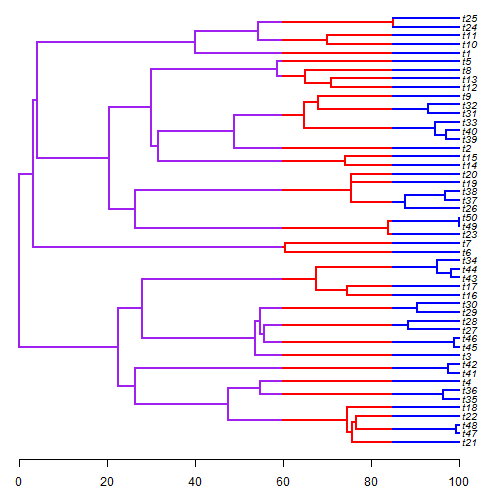Let's fit one, two, three, and four rate models to these data:

``````fit1<-rateshift(tree,x,nrates=1,niter=10)
``````
``````## Optimization progress:
## |..........|
## Done.
``````
``````fit1
``````
``````## ML 1-rate model:
##  s^2(1)  se(1)   k   logL
## value    7.4779  1.4956  2   -206.1193
##
## This is a one-rate model.
##
## Frequency of best fit: 1
##
## R thinks it has found the ML solution.
``````
``````fit2<-rateshift(tree,x,nrates=2,niter=10)
``````
``````## Optimization progress:
## |..........|
## Done.
``````
``````fit2
``````
``````## ML 2-rate model:
##  s^2(1)  se(1)   s^2(2)  se(2)   k   logL
## value    13.8711 3.3579  0.5263  0.2124  4   -193.311
##
## Shift point(s) between regimes (height above root):
##  1|2 se(1|2)
## value    88.4271 0.0436
##
## Frequency of best fit: 0.9
##
## R thinks it has found the ML solution.
``````
``````fit3<-rateshift(tree,x,nrates=3,niter=10)
``````
``````## Optimization progress:
## |..........|
## Done.
``````
``````fit3
``````
``````## ML 3-rate model:
##  s^2(1)  se(1)   s^2(2)  se(2)   s^2(3)  se(3)   k   logL
## value    7e-04   NaN 16.5352 4.7994  0.5158  0.2057  6   -190.4577
##
## Shift point(s) between regimes (height above root):
##  1|2 se(1|2) 2|3 se(2|3)
## value    48.8951 10.6356 88.4271 0.0387
##
## Frequency of best fit: 0.7
##
## R thinks it has found the ML solution.
``````
``````fit4<-rateshift(tree,x,nrates=4,niter=10)
``````
``````## Optimization progress:
## |..........|
## Done.
``````
``````fit4
``````
``````## ML 4-rate model:
##  s^2(1)  se(1)   s^2(2)  se(2)   s^2(3)  se(3)   s^2(4)  se(4)   k   logL
## value    7e-04   NaN 833.039 NaN 5.0879  2.2729  0.5437  0.2229  8   -188.5406
##
## Shift point(s) between regimes (height above root):
##  1|2 se(1|2) 2|3 se(2|3) 3|4 se(3|4)
## value    68.2645 NaN 69.0033 NaN 90.5243 0.07
##
## Frequency of best fit: 0.2
##
## R thinks it has found the ML solution.
``````
``````AIC(fit1,fit2,fit3,fit4)
``````
``````##      df      AIC
## fit1  2 416.2387
## fit2  4 394.6221
## fit3  6 392.9153
## fit4  8 393.0813
``````

We can plot our best model, in this case the generating, three-rate model:

``````plot(fit3,fsize=0.8)
``````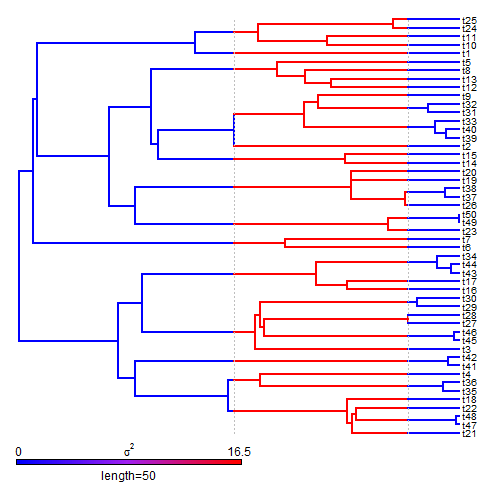Finally, I mentioned that it is possible to get trapped on local optima. This is true, somewhat surprisingly, even for the relatively simple, two-rate model. We can see why by visualizing the likelihood surface across the length of the tree:

``````shift<-1:99/100*max(nodeHeights(tree))
logL<-sapply(shift,function(s,tree,x) logLik(rateshift(tree,x,fixed.shift=s,
quiet=TRUE)),tree=tree,x=x)
plot(shift,logL,type="l",lwd=2)
plotTree(tree,color=rgb(0,0,1,0.25),ftype="off",mar=c(5.1,4.1,4.1,2.1),
``````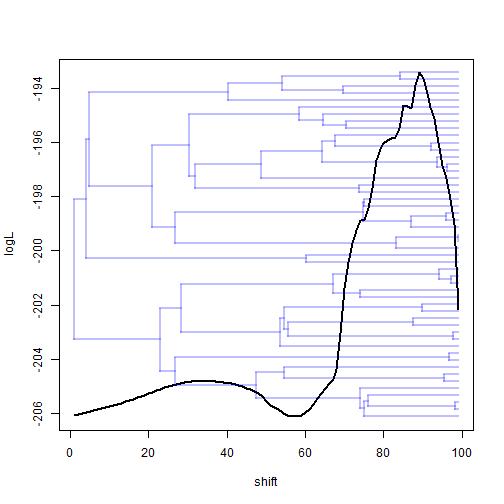We can also do this in two dimensions for the three-rate model:

``````shift<-seq(2,98,by=2)/100*max(nodeHeights(tree))
logL<-sapply(shift,function(s1,s2,tree,x)
sapply(s2,function(s2,s1,tree,x)
logLik(rateshift(tree,x,fixed.shift=if(s1!=s2) c(s1,s2) else s1,
quiet=TRUE)),s1=s1,tree=tree,x=x),s2=shift,tree=tree,x=x)
image(shift,shift,logL,col=gray.colors(60,0,1),xlab="shift 1 (or 2)",
ylab="shift 2 (or 1)")
points(fit3\$shift,fit3\$shift,pch=4) ## MLE
points(fit3\$shift,fit3\$shift,pch=4)
``````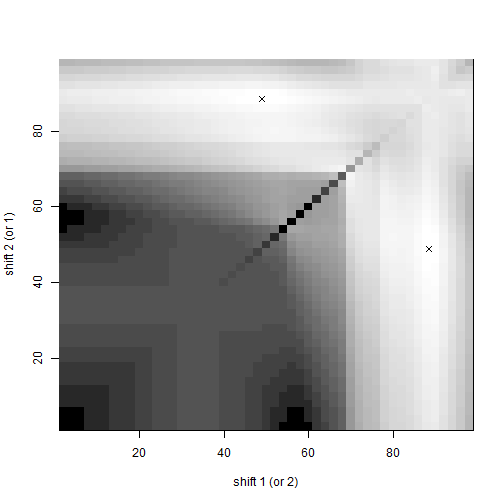Or, alternatively:

``````library(lattice)
wireframe(logL,row.values=shift,column.values=shift,xlab="shift 1 (or 2)",
ylab="shift 2 (or 1)",colorkey=TRUE,drape=TRUE)
``````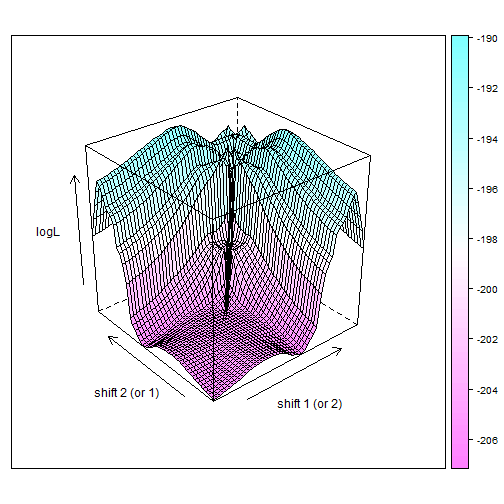Interesting!